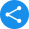# NCERT Class 9 Maths Book PDF

### NCERT Solutions for Class 9

1. How many chapters are there in Class 9 NCERT textbook?

A. There are a total of 15 chapters in the Class 9 NCERT textbook. Here the list is given in the following.

• Chapter 1 - Number Systems

• Chapter 2 - Polynomials

• Chapter 3 - Coordinate Geometry

• Chapter 4 - Linear Equations in Two Variables

• Chapter 5 - Introduction to Euclid’s Geometry

• Chapter 6 - Lines and Angles

• Chapter 7 - Triangles

• Chapter 9 - Areas of Parallelograms and Triangles

• Chapter 10 - Circles

• Chapter 11 - Constructions

• Chapter 12 - Heron’s Formula

• Chapter 13 - Surface Areas and Volumes

• Chapter 14 - Statistics

• Chapter 15 - Probability

2. Why is the Class 9 Maths NCERT Book important?

A. Class 9 Maths NCERT Book is well known for putting all the concepts in the simplest way possible so that the students can hold command over those very easily and quickly. That is why the NCERT book is considered as the best study material to excel in their studies. The importance of Class 9 Maths NCERT Book is mentioned in the following:

• The NCERT book for Class 9 Maths is designed to offer in-depth knowledge about all the concepts in easier and simple language.

• This textbook strictly adheres to the latest CBSE curriculum and guidelines due to which it is considered to be sufficient enough to build the strong fundamentals for the final exams.

• Class 9 Maths NCERT Book plays a crucial part in developing a clear concept as this provides a thorough and comprehensive knowledge.

• This book has a lot of exercises which contain several questions of different formats which are perfect to test students' understanding skills.

3. What is taught in Chapter 7 of Class 9 Maths NCERT Book?

A. Chapter 7 of Class 9 Maths NCERT Book titled Triangles deals with the triangle’s properties. Class 9 Maths students will learn about the congruence of triangles, criteria for congruence of triangles, properties of a triangle, and inequalities in a triangle through this chapter.

4. How can I download the PDF file of Class 9 Maths NCERT Book?

A. Yes, all the Class 9 students can download the CBSE board Class 9 Maths NCERT Solutions by clicking on the aforementioned link given on this page. Any student can download the PDF file of Class 9 Maths NCERT book very easily and that too at absolutely free of cost.

This book helps to lay a stronger foundation of the concepts. NCERT Book for class 9 Maths helps students by providing detailed knowledge to them about the most important topics and concepts under the Class 9th Maths syllabus.

Share this with your friendsShare